JEE  >  Sample JEE Main Full Mock Test

# Sample JEE Main Full Mock Test

Test Description

## 90 Questions MCQ Test | Sample JEE Main Full Mock Test

Sample JEE Main Full Mock Test for JEE 2023 is part of JEE preparation. The Sample JEE Main Full Mock Test questions and answers have been prepared according to the JEE exam syllabus.The Sample JEE Main Full Mock Test MCQs are made for JEE 2023 Exam. Find important definitions, questions, notes, meanings, examples, exercises, MCQs and online tests for Sample JEE Main Full Mock Test below.
Solutions of Sample JEE Main Full Mock Test questions in English are available as part of our course for JEE & Sample JEE Main Full Mock Test solutions in Hindi for JEE course. Download more important topics, notes, lectures and mock test series for JEE Exam by signing up for free. Attempt Sample JEE Main Full Mock Test | 90 questions in 180 minutes | Mock test for JEE preparation | Free important questions MCQ to study for JEE Exam | Download free PDF with solutions
 1 Crore+ students have signed up on EduRev. Have you?
Sample JEE Main Full Mock Test - Question 1

### The most stable measure of central tendency is

Detailed Solution for Sample JEE Main Full Mock Test - Question 1

Mean is the most frequently used measure of central tendency and generally considered the best measure of it. However, there are some situations where either median or mode are preferred. Median is the preferred measure of central tendency when: There are a few extreme scores in the distribution of the data.

Sample JEE Main Full Mock Test - Question 2

### The transition from the state n = 3 to n=1 in a hydrogen-like atom results in ultraviolet radiation. Infrared radiation will be obtained in the transition from.

Detailed Solution for Sample JEE Main Full Mock Test - Question 2

Infrared radiation is found in Paschen, Brackett and pfund series nd it is obtained when electron transition occurs from high energy level to minimum third level.

Sample JEE Main Full Mock Test - Question 3

### Eight drops of mercury of equal radii possessing equal charges combine to form a big drop. Then the capacitance of bigger drop compared to each individual small drop is

Detailed Solution for Sample JEE Main Full Mock Test - Question 3

Volume of 8 small drops = Volume of big drop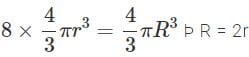As capacity is r, hence capacity becomes 2 times.

Sample JEE Main Full Mock Test - Question 4

If the body is moving in a circle of radius r with a constant speed υ, its angular velocity is

Detailed Solution for Sample JEE Main Full Mock Test - Question 4 In circular motion, angular velocity (w)= v( linear velocity) / radius if circle (r)
Sample JEE Main Full Mock Test - Question 5

To convert a 800 mV range milli voltmeter of resistance 40 Ω into a galvanometer of 100 mA range, the resistance to be connected as shunt is

Detailed Solution for Sample JEE Main Full Mock Test - Question 5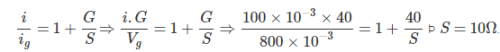Sample JEE Main Full Mock Test - Question 6

A point charge q is placed at the centre of a skeleton cube made of thin non-conducting wire. The electric flux passing through one face of the cube is

Detailed Solution for Sample JEE Main Full Mock Test - Question 6 Because total flux coming out from a closed body is q/ě. So flux through 1 face is 1/6th of it.
Sample JEE Main Full Mock Test - Question 7

The magnetic moment of a circular coil carrying current is

Detailed Solution for Sample JEE Main Full Mock Test - Question 7

u = NiA
Area is proportional to square of length. Hence B is correct.

Sample JEE Main Full Mock Test - Question 8

Consider the statement p: 'New Delhi is a city'. Which of the following is not negation of p?

Detailed Solution for Sample JEE Main Full Mock Test - Question 8

All the statements in (1), (3), and (4) are equivalent and each is the negation of p.

Sample JEE Main Full Mock Test - Question 9

The diameter of a cylinder is measured using a Vernier callipers with no zero error. It is found that the zero of the Vernier scale lies between 5.10 cm and 5.15 cm of the main scale. The Vernier scale has 50 divisions equivalent to 2.45 cm. The 24th division of the Vernier scale exactly coincides with one of the main scale divisions. The diameter of the cylinder is

Sample JEE Main Full Mock Test - Question 10

The natural frequency of a L-C circuit is equal to

Detailed Solution for Sample JEE Main Full Mock Test - Question 10 At resonance, X(L)=X(C)
It means that the inductive & capacitive reactances are equal.

Value of inductive reactance is given by–
X(L)=2πfL
And that of capacitive reactance is given by–
X(C)=1/(2πfC)

Therefore, at resonance–

2πfL=1/(2πfC)

f²=1/[(2πC)×(2πL)]

f²=1/(4π²LC)

f=1/[2π√(LC)] => Resonant Frequency f(R) or f(0)
Sample JEE Main Full Mock Test - Question 11

A coil of inductance 40 henry is connected in series with a resistance of 8 ohm and the combination is joined to the terminals of a 2 volt battery. The time constant of the circuit is

Detailed Solution for Sample JEE Main Full Mock Test - Question 11
Time constant in in RL circuit is t = L/R

L = 40 henry

R = 8 ohm

t = L/R = 40/8 = 5 second

It is the time in which the current in RL circuit grows to 63.2 % of its peak value.
Sample JEE Main Full Mock Test - Question 12

Proton and α-particles have the same de-Broglie wavelength. What is the same for both of them ?

Detailed Solution for Sample JEE Main Full Mock Test - Question 12 De brogile wavelength has formula lambda =h/MV. h is Planck's constant. If they have same de brogile wavelength then they have same m.v as h is constant.
Sample JEE Main Full Mock Test - Question 13

The energy released in a typical nuclear fusion reaction is approximately

Sample JEE Main Full Mock Test - Question 14

The angular velocity of the second needle in watch (in rad/s) is

Detailed Solution for Sample JEE Main Full Mock Test - Question 14
The seconds hand of a clock completes one rotation in 1 minute i.e 60 seconds.

Angular speed=angle swept by the radius vector/time taken

Angle swept for one complete rotation is 2π radians

Therefore, 2π/60=π/302π/60=π/30
Sample JEE Main Full Mock Test - Question 15

After an interval of one day, 1/16th of the initial amount of a radioactive material remains in a sample. It's half life will be

Detailed Solution for Sample JEE Main Full Mock Test - Question 15 If 1/16 of the initial material is left after a day or 24 hours means it has gone through 4 half lives. So the answer will be 24/4 = 6 hours.
Sample JEE Main Full Mock Test - Question 16

Two infinite plane parallel sheets, separated by a distance d have equal and opposite uniform charge densities σ. Electric field at a point between the sheets is

Sample JEE Main Full Mock Test - Question 17

A straight wire of length l and electric dipole moment p is bent to form a semicircle. The new dipole moment would be

Sample JEE Main Full Mock Test - Question 18

A block of mass 10 kg is placed on rough horizontal surface whose coefficient of friction is 0.5. If a horizontal force of 100 N is applied on it, then acceleration of block will be

Detailed Solution for Sample JEE Main Full Mock Test - Question 18

Friction = (0.5)mg = 50 N
Net force in horizontal dirn = 100 N - 50 N = 50N
acceleration= 50/10 = 5 m/s2

Sample JEE Main Full Mock Test - Question 19

A bird weighs 2 kg and is inside a closed cage of 1 kg. If it starts flying, then what is the weight of the bird and cage assembly

Detailed Solution for Sample JEE Main Full Mock Test - Question 19

When the bird flies, it pushes air down to balance its weight. So the weight of the bird and closed cage assembly remains unchanged.

Sample JEE Main Full Mock Test - Question 20

NAND gate is

Sample JEE Main Full Mock Test - Question 21

A particle of mass M and charge Q moving with velocity V → describes a circular path of radius R when subjected to a uniform transverse magnetic field of induction B. The work done by the field when the particle completes one full circle is

Detailed Solution for Sample JEE Main Full Mock Test - Question 21
When particle describes circular path in a magnetic field, its velocity is always perpendicular to the magnetic force?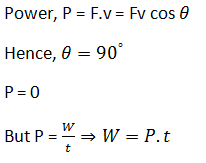Hence, work done W = 0 (everywhere)
Sample JEE Main Full Mock Test - Question 22

Which of the following is more effective in inducing nuclear fission

Sample JEE Main Full Mock Test - Question 23

If a liquid does not wet the glass, then its angle of contact is

Detailed Solution for Sample JEE Main Full Mock Test - Question 23

If a liquid does not wet the sides of containing vessel, then the value of angle of contact is obtuse i.e. greater than 90degree .e.g. mercury.

Sample JEE Main Full Mock Test - Question 24

The kinetic energy k of a particle moving along a circle of radius R depends on the distance covered s as K = as2 where a is a constant. The force acting on the particle is

Detailed Solution for Sample JEE Main Full Mock Test - Question 24

Given K.E = 1/2 mv2 = as2

= mv2 = 2as2

now differenciating with respect to t

2mv = dv/dt = 4as ds/dt = 4asv

mat = 2 as

Hence Ft = 2 as  here (Ft = tangential force)  .... 1>

centripetal force = Fc = mac = mv^2/R = 2 mas^2/ mR = 2as^2/R .... 2>

now net force of particle is = √Ft^2 + F^2 c = √(2as)^2 + (2as^2/R)^2

= 2 as(√1 + s^2/R^2or 2as(1 + s^2/R^2) raise to power 1/2

Sample JEE Main Full Mock Test - Question 25

A 1 kg stationary bomb exploded in three parts having mass ratio 1:1:3 . Parts having same mass are moving in perpendicular direction with velocity 30m/s, then the velocity of bigger part will be

Detailed Solution for Sample JEE Main Full Mock Test - Question 25

Conservation of momentum
1×0=1 ×30i +1×30j +3v
3v= -(30i +30j)
v = -(10i +10j)
v ( magnitude)= √(10)²+(10)²
=10√2 m/s

Sample JEE Main Full Mock Test - Question 26

Two identical masses of 5 kg each fall on a wheel from a height of 10 m . The wheel disturbs a mass of 22 kg water, the rise in temperature of water will be :-

Detailed Solution for Sample JEE Main Full Mock Test - Question 26

W = J Q

2m * g* h = J * m' * Cp * Delta T

2 *5*10*10 = 4.2 *(2* 1000* delta T)

Delta T = 0.12°c

Sample JEE Main Full Mock Test - Question 27

Dimension of CR are same as

Sample JEE Main Full Mock Test - Question 28

A parallel monochromatic beam of light is incident normally on a narrow slit. A diffraction pattern is formed on a screen placed perpendicular to the direction of the incident beam. At the first minimum of the diffraction pattern, the phase difference between the rays coming from the two edges of slit is

Detailed Solution for Sample JEE Main Full Mock Test - Question 28

The phase difference between the wavelets from the top and bottom edge of the slit=2π/λd sinθ
where d=width of slit
The first minimum of diffraction patterns occurs at,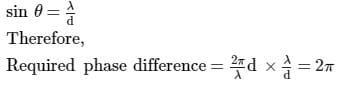Sample JEE Main Full Mock Test - Question 29

Both light and sound waves produce diffraction. It is more difficult to observe the diffraction with light waves because

Detailed Solution for Sample JEE Main Full Mock Test - Question 29 WAVELENGTH AND DIFFRACTION. The reason for the difference—that is, why sound diffraction is more pronounced than light diffraction—is that sound waves are much, much larger than light waves. Sound travels by longitudinal waves, or waves in which the movement of vibration is in the same direction as the wave itself.
Sample JEE Main Full Mock Test - Question 30

In Huygens wave theory, the locus of all points osculating in the same phase is called a

Detailed Solution for Sample JEE Main Full Mock Test - Question 30

Huygen, a Dutch mathematician, in 1678, gave a theory regarding nature of light. The theory is popularly known as Huygen’s wave theory. According to this theory, light is a sort of disturbance. The particles of the medium, vibrate in a direction, at right angle to the direction of propagation of disturbance. The process is called wave motion.

Straight wave front

Huygens's principle applied to a straight wave front. Each point on the wave front emits a semicircular wavelet that moves a distance s=vt. The new wavefront is a line tangent to the wavelets.

Sample JEE Main Full Mock Test - Question 31

The velocities of sound at the same temperature in two monoatomic gases of densities ρ₁ and ρ₂ are ν₁ and ν₂ respectively. If ρ₁/ρ₂= 4, then the value of ν₁/ν₂ is

Sample JEE Main Full Mock Test - Question 32

The alcohol which does not give a stable compound on dehydration is

Detailed Solution for Sample JEE Main Full Mock Test - Question 32

If you take out H2O from the Methyl Alcohol you will be left with CH2+ cation which is not stable.

Sample JEE Main Full Mock Test - Question 33

Vanaspati ghee is prepared by which of the following reactions of vegetable oils?

Detailed Solution for Sample JEE Main Full Mock Test - Question 33 Vanaspati ghee is made by hydrogenation of vegetable oils that is why it is considered harmful while vegetable oils are safe.
Sample JEE Main Full Mock Test - Question 34

Cinnamic acid is formed when C₆H₅CHO condenses with (CH₃CO)₂ in presence of

Sample JEE Main Full Mock Test - Question 35

According to Le-Chatelier's principle, adding heat to a solid and liquid in equilibrium will cause the

Detailed Solution for Sample JEE Main Full Mock Test - Question 35
When we add heat to the equilibrium between solid and liquid, then the equilibrium shifts towards liquid and hence, the amount of solid decrease and amount of liquid increase.

Sample JEE Main Full Mock Test - Question 36

A catalyst increases rate of reaction by

Detailed Solution for Sample JEE Main Full Mock Test - Question 36 A catalyst increases the rate of reaction by decreasing the energy of activation (Ea). a catalyst provides a new reaction path for the reaction. Because a catalyst forms an unstable intermediate with the reactants and it decomposes to give products and the catalyst is regenerated.
Sample JEE Main Full Mock Test - Question 37

C + O2 → C O2 , Δ H = − 94 K . c a l s ,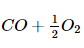→ C O2 , Δ H = − 67.7 K . cal .

On the basis of above data, the heat of formation of CO is

Detailed Solution for Sample JEE Main Full Mock Test - Question 37 Add equations 1 and (-2) and you'll see there's the formation of CO. So ∆H of formation of CO=(-94)-(-67.7)= -26.3 Kcal.
Sample JEE Main Full Mock Test - Question 38

Electron affinity depends on

Detailed Solution for Sample JEE Main Full Mock Test - Question 38

As the size of the atom increases, the electron affinity decreases, whereas when the nuclear charge increases the electron affinity increases.

Sample JEE Main Full Mock Test - Question 39

The type of isomerism present in nitropentaamminechromium (III) chloride is

Detailed Solution for Sample JEE Main Full Mock Test - Question 39 Nitropentamine chromium (III) chloride exhibits linkage isomerism as -NO2 is an ambidendate ligand.
Sample JEE Main Full Mock Test - Question 40

In the diazotisation of aniline with sodium nitrite and hydrochloric acid, the excess of hydrochloric acid is used primarily to

Detailed Solution for Sample JEE Main Full Mock Test - Question 40

An excess of HCl will suppress the hydrolysis of diazonium salt to phenol.

Sample JEE Main Full Mock Test - Question 41

On passing 0.1 faraday of electricity through fused sodium chloride, the amount of chloride liberated is (At. mass of Cl = 35.45)

Detailed Solution for Sample JEE Main Full Mock Test - Question 41 On passing 0.1F of electricity in NaCl, the amt of chloride liberated = 35.45*0.1 = 3.545
Sample JEE Main Full Mock Test - Question 42

The electrolysis of aqueous NaCl produces at cathode and anode respectively

Detailed Solution for Sample JEE Main Full Mock Test - Question 42 Electrolysis of aqueous sodium chloride: Electrolysis of aqueous NaCl results in hydrogen and chloride gas. At the anode (A), chloride (Cl-) is oxidized to chlorine. The ion-selective membrane (B) allows the counterion Na+ to freely flow across, but prevents anions such as hydroxide (OH-) and chloride from diffusing across. At the cathode (C), water is reduced to hydroxide and hydrogen gas. The net process is the electrolysis of an aqueous solution of NaCl into industrially useful products sodium hydroxide (NaOH) and chlorine gas.
Sample JEE Main Full Mock Test - Question 43

In which of the following acid-base titration, pH is greater at eqivalence point?

Sample JEE Main Full Mock Test - Question 44

Why are strong acids generally used as standard solutions in acid-base titrations ?

Sample JEE Main Full Mock Test - Question 45

If H⁺ ion conc. of a solution is increased by 10 times, its pH will

Detailed Solution for Sample JEE Main Full Mock Test - Question 45 Concept: pH = -log (H+)

If its concentration is increased 10 times its
pH = - log (10) = -1.
so it decreases by 1.

Sample JEE Main Full Mock Test - Question 46

When NaCl is added to the reaction mixture of an oil and caustic soda , the soap is thrown out because

Detailed Solution for Sample JEE Main Full Mock Test - Question 46

Formation of soap involves reaction with ester and NaOH when NaCl has added it results in the increase in repulsion between the ions namely NA+ which shifts the reaction in the formation of soap.

Sample JEE Main Full Mock Test - Question 47

Increasing order of pka values (pKa = - log ka ) of H2O , C H3OH and C6H5OH is :

Detailed Solution for Sample JEE Main Full Mock Test - Question 47

Option d is correct. Methanol is more acidic than water. So its pka value will be less than water. However C5H5OH wll be most acidic among all three.SO he order of pka is C6H5OH < CH3OH < H2O

Sample JEE Main Full Mock Test - Question 48

The compound which can form intramolecular hydrogen bond is

Sample JEE Main Full Mock Test - Question 49

Which one is a polymer compound?

Detailed Solution for Sample JEE Main Full Mock Test - Question 49

Pvc is polymer compound and many other compound that made plastic are called polymer compound while pvc is present in plastic bottle.

Sample JEE Main Full Mock Test - Question 50

Ionic solids with Schottky defects contain in their structure

Sample JEE Main Full Mock Test - Question 51

Diamond is a

Sample JEE Main Full Mock Test - Question 52

If 107 g of an aqueous solution contains 39.5 g of a solute (mol. weight = 158), then mole fraction of the solute is

Detailed Solution for Sample JEE Main Full Mock Test - Question 52 First find no. of moles of solute that is 0.25(39.5/158)then moles of solvent that is 107-39.5/18 = 3.75 mole reaction of solute = no of moles of solute/total moles 0.25/4 into 100 = 6.25
Sample JEE Main Full Mock Test - Question 53

Which of the following compound corresponds Vant's Hoff factor (i) to be equal to 2 in a dilute solution?

Detailed Solution for Sample JEE Main Full Mock Test - Question 53

This is because, it will dissociate into Mg2+ and SO42-, i.e. two ions.

Sample JEE Main Full Mock Test - Question 54

One mole of 1, 2-Dibromopropane on treatment with X moles of NaNH₂ followed by treatment with ethyl bromide gave a pentyne. The value of X is

Detailed Solution for Sample JEE Main Full Mock Test - Question 54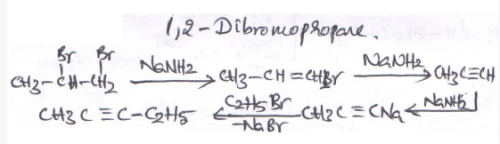Sample JEE Main Full Mock Test - Question 55

Ethyl bromide on treatment with alcoholic KOH gives

Sample JEE Main Full Mock Test - Question 56

If the energy of an electron in the second energy level of hydrogen atom is -E, its value in the third energy level will be __

Detailed Solution for Sample JEE Main Full Mock Test - Question 56

According to question :
− E = -13.6 x Z2 /n2
o r − 13.6 = − E x 4 (i)
Energy for third energy level:
E3 = − 13.6 x Z2 32
E3 x 9 = − 13.6 i i
By (i) and (ii)
− E x 4 = E 3 x 9
or E3 = − 4E /9

Sample JEE Main Full Mock Test - Question 57

Suppose 10-17 J of energy is needed by the interior of human eye of to see an object. How many photons of green light (λ = 500 nm) are needed to generate this minimum amount of energy?

Detailed Solution for Sample JEE Main Full Mock Test - Question 57

Let the number of photons required be n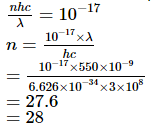Sample JEE Main Full Mock Test - Question 58

Which of the following is correct relation of first law of thermodynamics ?

Sample JEE Main Full Mock Test - Question 59

An activated complex molecule differs from a normal molecule of the same atomicity in that it has__

Sample JEE Main Full Mock Test - Question 60

Which of the following ions has zero crystal field stabilization energy in octahedral field?

Sample JEE Main Full Mock Test - Question 61

An organic compound with C = 40% and H = 6.7% will have the empirical formula

Detailed Solution for Sample JEE Main Full Mock Test - Question 61

C is 40% and H is 6.7% so O is 53.3%
now calculate % / at. weight
c= 40/12
H=6.7/1
O=53.3/16
now get the simplest ratio as CH2O

Sample JEE Main Full Mock Test - Question 62

For which one of the following values of k, the equation
2x3 + 3x2 − 12x − k = 0 has three distinct real roots?

Sample JEE Main Full Mock Test - Question 63

If xh yk is an integrating factor of the differential equation
y(1 + xy) dx + x(1 − xy) dy = 0,
then the ordered pair (h, k) is equal to

Sample JEE Main Full Mock Test - Question 64

Let A be the square of natural numbers and x, y are any two elements of A. Then

Detailed Solution for Sample JEE Main Full Mock Test - Question 64

Because as x is a squared quantity and y is also a squared quantity so xy will also be perfect square of a number but in case of x+y and x-y it may be perfect square or not.

Sample JEE Main Full Mock Test - Question 65

If 'n' is a positive integer, then n3 + 2n is divisible by

Sample JEE Main Full Mock Test - Question 66

The area enclosed between the parabola y2 = 4ax and the lines x = a, x = 9a is

Sample JEE Main Full Mock Test - Question 67

Area of the curve x2+y2=2ax is

Detailed Solution for Sample JEE Main Full Mock Test - Question 67

It is a circle whose equation is (x-a)2+y2=a2

with radius a. So area of such a circle is πa2

Sample JEE Main Full Mock Test - Question 68

The equation of the circle passing through (0,0),(0,a),(a,0) is

Detailed Solution for Sample JEE Main Full Mock Test - Question 68
The general equation of circle is x^2+y^2+2gx+2fy+c=0 where center is (-g,-f)

Given the point (0,0 ) Equation of the circle through that point is c=0

Given the point (a,0).Equation of the circle through that point is a^2+2ga+c=0

⇒a^2+2ag=0

a(a+2g)=0→g=−a2⇒g=−a/2

Given the point (0,a)  Equation of the circle through that point is a^2+2fa+c=0

⇒a^2+2fa=0→a(a+2f)=0→a=-2f

f=-a/2

the equation will be

x^2+y^2+2(-a/2)x+2(-a/2)y+0=0

x^2+y^2-ax-ay=0

x^2+y^2-a(x+y)=0

Sample JEE Main Full Mock Test - Question 69

[(1 + sinθ + icosθ)/(1 +sinθ - icosθ)]n =

Sample JEE Main Full Mock Test - Question 70

If the imaginary part of [(2z+1)/(iz+1)] is -2, then the locus of z in complex plane represent.

Sample JEE Main Full Mock Test - Question 71

Solution of the differential equation tan y sec2 x dx + tan x sec2 y dy = 0 is

Sample JEE Main Full Mock Test - Question 72

(d/dx){log(secx+tanx)}=

Detailed Solution for Sample JEE Main Full Mock Test - Question 72

d/dx {log Sec x + tan x}
= 1 Sec x + tan x Sec x . tan x + sec 2x
= Sec x Sec x + tan x Sec x + tan x
= Sec x

Sample JEE Main Full Mock Test - Question 73

If f (x)   =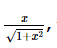, then f of f(x) =

Detailed Solution for Sample JEE Main Full Mock Test - Question 73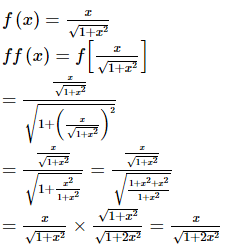Sample JEE Main Full Mock Test - Question 74

When y = 3, which of the following is FALSE?

Sample JEE Main Full Mock Test - Question 75

If A is a non-zero column matrix of order m x1 and B is a non-zero row matrix of order 1 x n, then rank of AB is equal to

Detailed Solution for Sample JEE Main Full Mock Test - Question 75

Let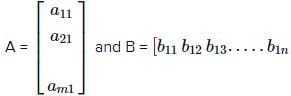be two non-zero column and row matrices respectively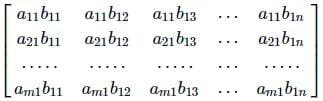Since A, B are non-zero matrices. .% matrix AB will be a non-zero matrix. The matrix AB will have at least one non-zero element obtained by multiplying corresponding non-zero elements of A and B. All the two rowed minors of AB clearly vanish. Since AB is non-zero matrix,
∴ rank of AB = 1

Sample JEE Main Full Mock Test - Question 76

The line y=mx+c touches the parabola x2=4ay if

Detailed Solution for Sample JEE Main Full Mock Test - Question 76

Concept: Straight line. x2=4ay.............(1)
y=mx+c....,........(2)
From (1) and (2) we get x2 = 4a(mx+c)
=> x2−4amx−4ac=0
=16a2m2+16ac(b2 -4ac). =16a(am2 + c ).
Line 2 will touch the parabola. if D=0. i.e if C=−am2

Sample JEE Main Full Mock Test - Question 77

Number of ways in which 6 persons can be seated around a table so that two particular persons are never seated together is equal to

Detailed Solution for Sample JEE Main Full Mock Test - Question 77

5! - 2×4! =72

Sample JEE Main Full Mock Test - Question 78

Two dice are thrown simultaneously. What is the probability of getting two numbers whose product is even?

Detailed Solution for Sample JEE Main Full Mock Test - Question 78

In a simultaneous throw of two dice, we have n(S) = (6 x 6) = 36.
Then, E = ((1, 2), (1, 4), (1, 6), (2, 1), (2, 2), (2, 3), (2, 4), (2, 5), (2, 6), (3, 2), (3, 4), (3, 6), (4, 1), (4, 2), (4, 3), (4, 4), (4, 5), (4, 6), (5, 2), (5, 4), (5, 6), (6, 1), (6, 2), (6, 3), (6, 4), (6, 5), (6, 6)}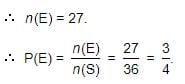Sample JEE Main Full Mock Test - Question 79

The equation of a line passing through (-4,3) and this point divides the portion of line between axes in the ratio 5:3 internally, is

Detailed Solution for Sample JEE Main Full Mock Test - Question 79

Let the line through the point P(-A, 3) meets axis at A(h, 0) and 0(0, k)
Now according to the question AP : BP =5:3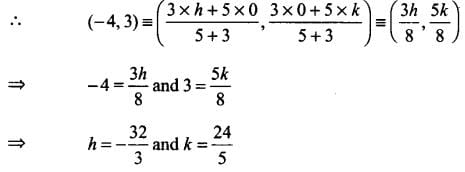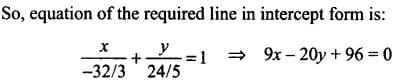Sample JEE Main Full Mock Test - Question 80

The length of the sub normal to the parabola y2=4ax at any point is equal to

Detailed Solution for Sample JEE Main Full Mock Test - Question 80 The length of the subnormal is always constant and is equal to the semi-latus rectum i.e. 2a …Hence D is the correct.
Sample JEE Main Full Mock Test - Question 81

The locus of x2+y2+z2=0 is

Sample JEE Main Full Mock Test - Question 82

cos2α+cos2(α+120º)+cos2(α-120º)=

Detailed Solution for Sample JEE Main Full Mock Test - Question 82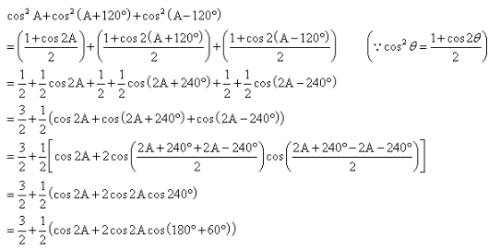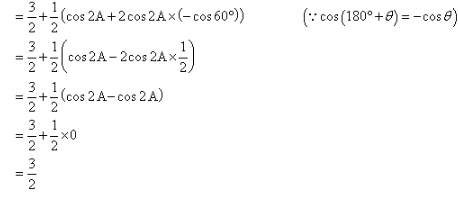Sample JEE Main Full Mock Test - Question 83

If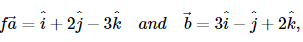, then the angle between the vectors a → + b → and a → − b →

is

Sample JEE Main Full Mock Test - Question 84

The points with position vectors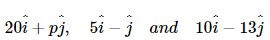are collinear. The value of p is

Sample JEE Main Full Mock Test - Question 85

In the following question, a Statement of Assertion (A) is given followed by a corresponding Reason (R) just below it. Read the Statements carefully and mark the correct answer-
Assertion(A): At x = 0, the curve y = 11 + x2 has the greatest slope.
Reason(R) : dy/dx = 0 and d2 + yd x2 < 0 at x = 0

Sample JEE Main Full Mock Test - Question 86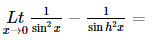Sample JEE Main Full Mock Test - Question 87

If A is a singular matrix, then A adj (A)

Sample JEE Main Full Mock Test - Question 88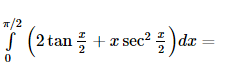Detailed Solution for Sample JEE Main Full Mock Test - Question 88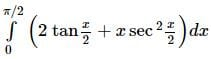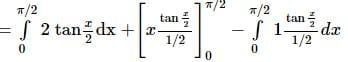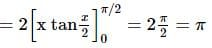Sample JEE Main Full Mock Test - Question 89

Let α,β be the roots of x2-x+p=0 and γ,δ be the roots of x2-4x+q=0. If α,β,γ,δ are in G.P., then the integer values of p and q respectivley are :

Detailed Solution for Sample JEE Main Full Mock Test - Question 89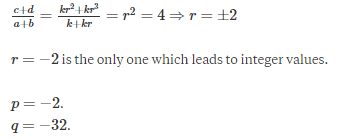Sample JEE Main Full Mock Test - Question 90

If f(x)=x5-5x4+5x3-10 how local max and min at x=p and x=q respectively, then (p,q)=

 Use Code STAYHOME200 and get INR 200 additional OFF Use Coupon Code
Information about Sample JEE Main Full Mock Test Page
In this test you can find the Exam questions for Sample JEE Main Full Mock Test solved & explained in the simplest way possible. Besides giving Questions and answers for Sample JEE Main Full Mock Test, EduRev gives you an ample number of Online tests for practice